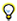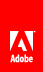# Miscellaneous optimizationsFor a TextField object, use the appendText() method instead of the += operator. When working with the text property of the TextField class, use the appendText() method instead of the += operator. Using the appendText() method provides performance improvements. As an example, the following code uses the += operator, and the loop takes 1120 ms to complete: ```addChild ( myTextField );   myTextField.autoSize = TextFieldAutoSize.LEFT; var started:Number = getTimer();   for (var i:int = 0; i< 1500; i++ ) { myTextField.text += "ActionScript 3"; }   trace( getTimer() - started ); // output : 1120 ``` In the following example, the += operator is replaced with the appendText() method: ```var myTextField:TextField = new TextField(); addChild ( myTextField ); myTextField.autoSize = TextFieldAutoSize.LEFT;   var started:Number = getTimer();   for (var i:int = 0; i< 1500; i++ ) { myTextField.appendText ( "ActionScript 3" ); } trace( getTimer() - started ); // output : 847 ``` The code now takes 847 ms to complete.Update text fields outside loops, when possible. This code can be optimized even further by using a simple technique. Updating the text field in each loop uses much internal processing. By simply concatenating a string and assigning it to the text field outside the loop, the time to run the code drops substantially. The code now takes 2 ms to complete: ```var myTextField:TextField = new TextField(); addChild ( myTextField ); myTextField.autoSize = TextFieldAutoSize.LEFT; var started:Number = getTimer(); var content:String = myTextField.text;   for (var i:int = 0; i< 1500; i++ ) { content += "ActionScript 3"; }   myTextField.text = content;   trace( getTimer() - started ); // output : 2 ``` When working with HTML text, the former approach is so slow that it can throw a Timeout exception in Flash Player, in some cases. For example, an exception can be thrown if the underlying hardware is too slow. Note: Adobe® AIR® does not throw this exception. ```var myTextField:TextField = new TextField(); addChild ( myTextField ); myTextField.autoSize = TextFieldAutoSize.LEFT;   var started:Number = getTimer();   for (var i:int = 0; i< 1500; i++ ) { myTextField.htmlText += "ActionScript 2"; } trace( getTimer() - started ); ``` By assigning the value to a string outside the loop, the code requires only 29 ms to complete: ```var myTextField:TextField = new TextField(); addChild ( myTextField ); myTextField.autoSize = TextFieldAutoSize.LEFT;   var started:Number = getTimer(); var content:String = myTextField.htmlText;   for (var i:int = 0; i< 1500; i++ ) { content += "ActionScript 3"; }   myTextField.htmlText = content;   trace ( getTimer() - started ); // output : 29 ``` Note: In Flash Player 10.1 and AIR 2.5, the String class has been improved so that strings use less memory.Avoid using the square bracket operator, when possible. Using the square bracket operator can slow down performance. You can avoid using it by storing your reference in a local variable. The following code example demonstrates inefficient use of the square bracket operator: ```var lng:int = 5000; var arraySprite:Vector. = new Vector.(lng, true); var i:int; for ( i = 0; i< lng; i++ ) { arraySprite[i] = new Sprite(); } var started:Number = getTimer(); for ( i = 0; i< lng; i++ ) { arraySprite[i].x = Math.random()*stage.stageWidth; arraySprite[i].y = Math.random()*stage.stageHeight; arraySprite[i].alpha = Math.random(); arraySprite[i].rotation = Math.random()*360; } trace( getTimer() - started ); // output : 16 ``` The following optimized version reduces the use of the square bracket operator: ```var lng:int = 5000; var arraySprite:Vector. = new Vector.(lng, true); var i:int; for ( i = 0; i< lng; i++ ) { arraySprite[i] = new Sprite(); } var started:Number = getTimer(); var currentSprite:Sprite; for ( i = 0; i< lng; i++ ) { currentSprite = arraySprite[i]; currentSprite.x = Math.random()*stage.stageWidth; currentSprite.y = Math.random()*stage.stageHeight; currentSprite.alpha = Math.random(); currentSprite.rotation = Math.random()*360; } trace( getTimer() - started ); // output : 9 ```Inline code, when possible, to reduce the number of function calls in your code. Calling functions can be expensive. Try to reduce the number of function calls by moving code inline. Moving code inline is a good way to optimize for pure performance. However, keep in mind that inline code can make your code harder to reuse and can increase the size of your SWF file. Some function calls, such the Math class methods, are easily to move inline. The following code uses the Math.abs() method to calculate absolute values: ```const MAX_NUM:int = 500000; var arrayValues:Vector.=new Vector.(MAX_NUM,true); var i:int;   for (i = 0; i< MAX_NUM; i++) { arrayValues[i] = Math.random()-Math.random(); }   var started:Number = getTimer(); var currentValue:Number;   for (i = 0; i< MAX_NUM; i++) { currentValue = arrayValues[i]; arrayValues[i] = Math.abs ( currentValue ); }   trace( getTimer() - started ); // output : 70``` The calculation performed by Math.abs() can be done manually and moved inline: ```const MAX_NUM:int = 500000; var arrayValues:Vector.=new Vector.(MAX_NUM,true); var i:int;   for (i = 0; i< MAX_NUM; i++) { arrayValues[i] = Math.random()-Math.random(); }   var started:Number = getTimer(); var currentValue:Number;   for (i = 0; i< MAX_NUM; i++) { currentValue = arrayValues[i]; arrayValues[i] = currentValue > 0 ? currentValue : -currentValue; }   trace( getTimer() - started ); // output : 15``` Moving the function call inline results in code that is more than four times faster. This approach is useful in many situations, but be aware of the effect it can have on reusability and maintainability. Note: Code size has a large impact on overall player execution. If the application includes large amounts of ActionScript code, then the virtual machine spends significant amounts of time verifying code and JIT compiling. Property lookups can be slower, because of deeper inheritance hierarchies and because internal caches tend to thrash more. To reduce code size, avoid using the Adobe® Flex® framework, the TLF framework library, or any large third-party ActionScript libraries.Avoid evaluating statements in loops. Another optimization can be achieved by not evaluating a statement inside a loop. The following code iterates over an array, but is not optimized because the array length is evaluated for each iteration: ```for (var i:int = 0; i< myArray.length; i++) { }``` It is better to store the value and reuse it: ```var lng:int = myArray.length; for (var i:int = 0; i< lng; i++) { }```Use reverse order for while loops. A while loop in reverse order is faster than a forward loop: ```var i:int = myArray.length; while (--i > -1) { }``` These tips provide a few ways to optimize ActionScript, showing how a single line of code can affect performance and memory. Many other ActionScript optimizations are possible. For more information, see the following link: http://www.rozengain.com/blog/2007/05/01/some-actionscript-30-optimizations/ .Twitter™ and Facebook posts are not covered under the terms of Creative Commons.// Ethnio survey code removed